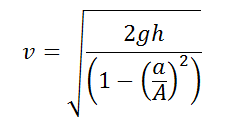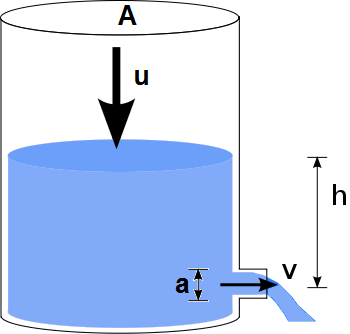# Velocity of Efflux using Torricelli's theorem

This CalcTown calculator calculates the velocity of liquid efflux from a narrow hole at the bottom of a container, using Torricell's principle.

*Please enter a=0 and A=1 if the condition A>>a is given.

m
m2
m2

#### Result

m/sClick here to view image

where

a=Cross-Sectional area of the hole

A=Cross-Sectional area of the top portion

h=Difference of height between the hole and the top surface

v=Velocity of the Efflux

u=Downward Velocity of the free surface